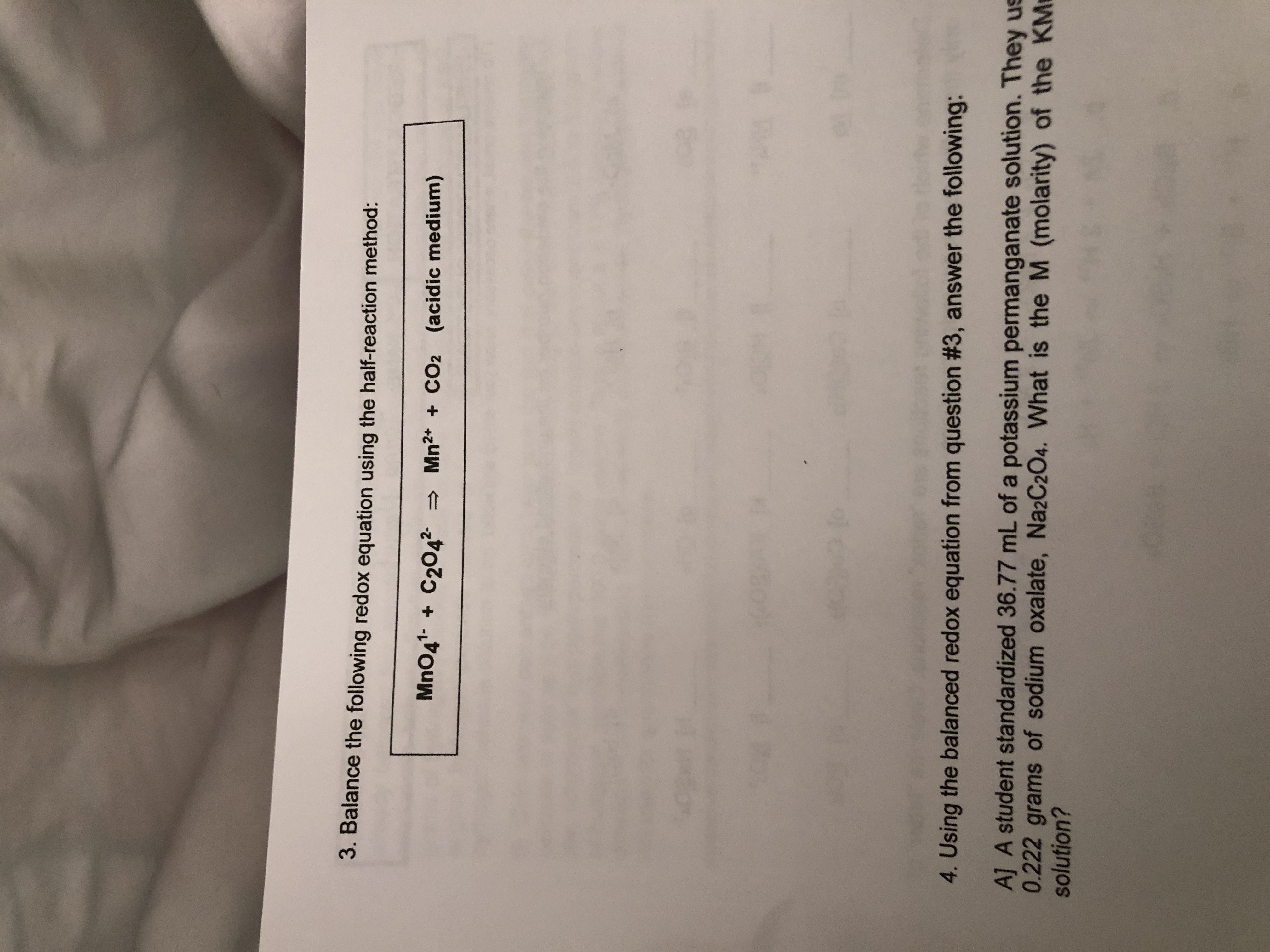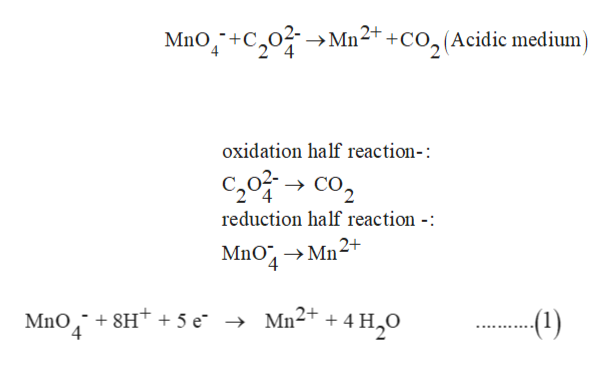# 3. Balance the following redox equation using the half-reaction method:MnO41 C2042 Mn2* +CO2 (acidic medium)4. Using the balanced redox equation from question #3, answer the following:A] A student standardized 36.77 mL of a potassium permanganate solution. They us0.222 grams of sodium oxalate, Na2C2O4. What is the M (molarity) of the KMsolution?

Question

Question 3help_outlineImage Transcriptionclose3. Balance the following redox equation using the half-reaction method: MnO41 C2042 Mn2* + CO2 (acidic medium) 4. Using the balanced redox equation from question #3, answer the following: A] A student standardized 36.77 mL of a potassium permanganate solution. They us 0.222 grams of sodium oxalate, Na2C2O4. What is the M (molarity) of the KM solution? fullscreen
check_circle

Step 1

Balancing the redox reaction by using the half-reaction method (acidic medium).

In this method, some point is followed during balancing the redox reaction.

1) Identify reduction -half and oxidation half.

2) In both half-reaction balance all the other atom, except oxygen and hydrogen atom.

3) Balance the oxygen atom by adding H2O.

4) Balance the hydrogen atom by adding H+ ion.

5) balance charge only by adding electrons.

Step 2

Since the given reaction is-

Balancing of reduction half-reaction

1) Since on both sides, Mn is one so it is balanced.

2) on the left-hand side Oxygen atom is 4 and in right- hand side oxygen atom is absent, so add 4 H2O in the right-side. Now to balance hydrogen atom add 8 H+ ions on the left side.

3)now on the left side total charge is +7 whereas on the right side is only+2. So to balance charge add 5 electrons on the left side.

Thus the balanced reduction half-reactionhelp_outlineImage TranscriptioncloseMno+C0M22+ +CO,(Acidic medium oxidation half reaction-: C20C reduction half reaction -: MnO MnO 8Ht + 5 e 4 - Mn2+ + 4 -(1) fullscreen
Step 3

Balancing of the oxidation half-reaction-:

1) on the left side 2 carbon atoms are present so to balance the carbon atom on right side multiply CO2 by 2

2) now on both side oxygen atom are...

### Want to see the full answer?

See Solution

#### Want to see this answer and more?

Solutions are written by subject experts who are available 24/7. Questions are typically answered within 1 hour.*

See Solution
*Response times may vary by subject and question.
Tagged in

### Chemistry